Kirchhoff’s Voltage Law

# Kirchhoff’s Voltage Law - Notes | Study Network Theory (Electric Circuits) - Electrical Engineering (EE)

 1 Crore+ students have signed up on EduRev. Have you?

Introduction

• Gustav Kirchhoff’s Voltage Law is the second of his fundamental laws we can use for circuit analysis. His voltage law states that for a closed loop series path the algebraic sum of all the voltages around any closed loop in a circuit is equal to zero. This is because a circuit loop is a closed conducting path so no energy is lost.
• In other words the algebraic sum of ALL the potential differences around the loop must be equal to zero as: ΣV = 0. Note here that the term “algebraic sum” means to take into account the polarities and signs of the sources and voltage drops around the loop.
• This idea by Kirchhoff is commonly known as the Conservation of Energy, as moving around a closed loop, or circuit, you will end up back to where you started in the circuit and therefore back to the same initial potential with no loss of voltage around the loop. Hence any voltage drops around the loop must be equal to any voltage sources met along the way.
• So when applying Kirchhoff’s voltage law to a specific circuit element, it is important that we pay special attention to the algebraic signs, (+ and –) of the voltage drops across elements and the emf’s of sources otherwise our calculations may be wrong.
• But before we look more closely at Kirchhoff’s voltage law (KVL) lets first understand the voltage drop across a single element such as a resistor.

A Single Circuit Element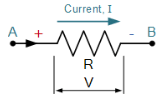• For this simple example we will assume that the current, I is in the same direction as the flow of positive charge, that is conventional current flow.
• Here the flow of current through the resistor is from point A to point B, that is from positive terminal to a negative terminal. Thus as we are travelling in the same direction as current flow, there will be a fall in potential across the resistive element giving rise to a -IR voltage drop across it.
• If the flow of current was in the opposite direction from point B to point A, then there would be a rise in potential across the resistive element as we are moving from a – potential to a + potential giving us a +I*R voltage drop.
• Thus to apply Kirchhoff’s voltage law correctly to a circuit, we must first understand the direction of the polarity and as we can see, the sign of the voltage drop across the resistive element will depend on the direction of the current flowing through it. As a general rule, you will loose potential in the same direction of current across an element and gain potential as you move in the direction of an emf source.
• The direction of current flow around a closed circuit can be assumed to be either clockwise or anticlockwise and either one can be chosen. If the direction chosen is different from the actual direction of current flow, the result will still be correct and valid but will result in the algebraic answer having a minus sign.

A Single Circuit Loop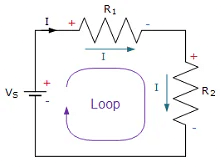• Kirchhoff’s voltage law states that the algebraic sum of the potential differences in any loop must be equal to zero as: ΣV = 0. Since the two resistors, R1 and R2 are wired together in a series connection, they are both part of the same loop so the same current must flow through each resistor.
• Thus the voltage drop across resistor, R1 = I*R1 and the voltage drop across resistor, R2 = I*R2 giving by KVL:
VS + (-IR1) + (-IR2) = 0
∴ V= IR1 + IR2
VS = I(R1 + R2)
VS = IRT
Where: RT = R1 + R2
• We can see that applying Kirchhoff’s Voltage Law to this single closed loop produces the formula for the equivalent or total resistance in the series circuit and we can expand on this to find the values of the voltage drops around the loop.
RT = R1 + R2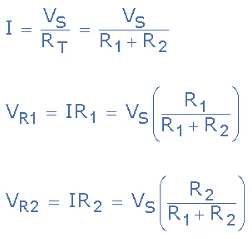Kirchhoff’s Voltage Law Example

• Three resistor of values: 10 ohms, 20 ohms and 30 ohms, respectively are connected in series across a 12 volt battery supply. Calculate: a) the total resistance, b) the circuit current, c) the current through each resistor, d) the voltage drop across each resistor, e) verify that Kirchhoff’s voltage law, KVL holds true.

(a) Total Resistance (RT)

RT = R1 + R2 + R3  =  10Ω + 20Ω + 30Ω = 60Ω
Then the total circuit resistance RT is equal to 60Ω

(b) Circuit Current (I)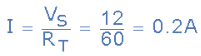Thus the total circuit current I is equal to 0.2 amperes or 200mA
(c) Current Through Each Resistor

The resistors are wired together in series, they are all part of the same loop and therefore each experience the same amount of current. Thus:

• IR= IR2 = IR3 = ISERIES  =  0.2 amperes

(d) Voltage Drop Across Each Resistor

• VR1 = I x R1 = 0.2 x 10  =  2 volts
• VR2 = I x R2 = 0.2 x 20  =  4 volts
• VR3 = I x R3 = 0.2 x 30  =  6 volts

(e) Verify Kirchhoff’s Voltage Law
VS + (-IR1) + (-IR2) + (-IR3)= 0
12 + (-0.2 X 10) + (-0.2 X 20) + (-0.2 X 30) = 0
12 + (-2) + (-4) + (-6) = 0
∴ 12 - 2 - 4 - 6 = 0
Thus Kirchhoff’s voltage law holds true as the individual voltage drops around the closed loop add up to the total.

Kirchhoff’s Circuit Loop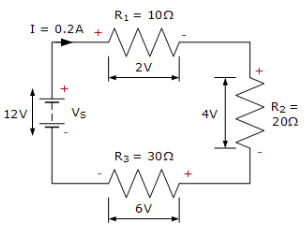•  We have seen here that Kirchhoff’s voltage law, KVL is Kirchhoff’s second law and states that the algebraic sum of all the voltage drops, as you go around a closed circuit from some fixed point and return back to the same point, and taking polarity into account, is always zero. That is ΣV = 0
The document Kirchhoff’s Voltage Law - Notes | Study Network Theory (Electric Circuits) - Electrical Engineering (EE) is a part of the Electrical Engineering (EE) Course Network Theory (Electric Circuits).
All you need of Electrical Engineering (EE) at this link: Electrical Engineering (EE)

## Network Theory (Electric Circuits)

23 videos|63 docs|60 tests
 Use Code STAYHOME200 and get INR 200 additional OFF

## Network Theory (Electric Circuits)

23 videos|63 docs|60 tests

Track your progress, build streaks, highlight & save important lessons and more!

,

,

,

,

,

,

,

,

,

,

,

,

,

,

,

,

,

,

,

,

,

;